#Streamline User Guide

### Site Tools

understanding-the-ir-calculation

# 6.4. Understanding Purchase Plan and Projected Inventory Levels

In this article, we explain how Streamline calculates:

To explain the calculations we will use the example project.

## Order now quantity

To explain, how the Order now qty column is calculated, let’s show the Demand forecast section of the inventory report table. To do this, go to the report settings and check the Demand forecast option (see figure below).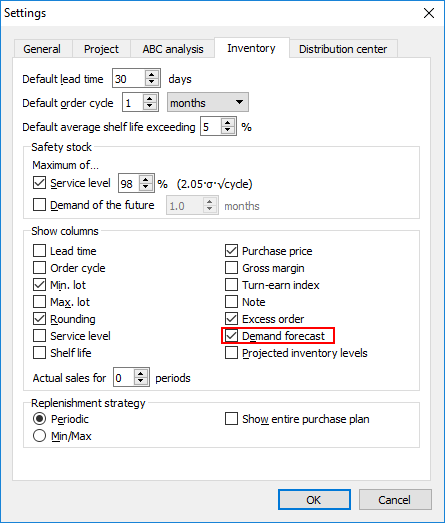Let us take a look at item H8010. The Order cycle and the Lead time are both two months. (see figure below).

The On hand is 105 units. That is enough to fulfill the first part of the lead time period (the forecast shows 94 units demand in January) but not enough to satisfy the last part of the lead time (the forecast is 91 units in February).

Streamline uses a color-coding for the On hand column and Demand forecast section of the report. In our case, a red background of the On hand cell means that there is currently insufficient inventory to cover the demand during the lead time period.

All we can do is to replenish the next order cycle period (March and April). Thus, Streamline suggests an order of 200 units currently to cover the demand during this period.

The formula for the Order now qty in Excel-like format is:

`Order now qty = MAX(CEILING(MAX(0, Order cycle demand [starting from Lead time] + Safety stock + Pending sales orders - Remaining), Rounding), Min lot)`, (1)

where:

`Remaining = MAX(0, MAX(0, On hand) + Qty to receive - Lead time demand)`.

Past due In transitions compensate negative On hand. It means that if you have past due Qty to receive of 100 units, On hand is -20, and Lead time demand is 40, then Remaining = MAX(0, -20 + 100 - 40) = 40.
Order now qty is rounded to Rounding if it exceeds Min lot, otherwise is replaced with the Min lot.

Let’s calculate the order quantity for our example. As we don’t have constraints for this item, the formula is simpler:

`Order now qty = Order cycle demand [starting from lead time] + Safety stock + Pending sales orders – Remaining`.

In our case:

Remaining = MAX(0, 105 + 0 – (94 + 91)) = 0.

Order now qty = (102 + 94) + 4 + 0 – 0 = 200.

## Purchase Plan and Projected Inventory Levels

In this section, we describe:

• the horizon of Streamline’s purchase plan and projected inventory levels;
• the data required for calculation a purchase plan;
• how a purchase plan and projected inventory levels are calculated by Streamline.

### Horizon of Purchase Plan and Projected Inventory Levels

Let’s go to the Inventory planning tab and show the Purchase plan, Projected inventory levels, and Demand forecast sections. To do this, click the Settings button and check the options shown in the figure below.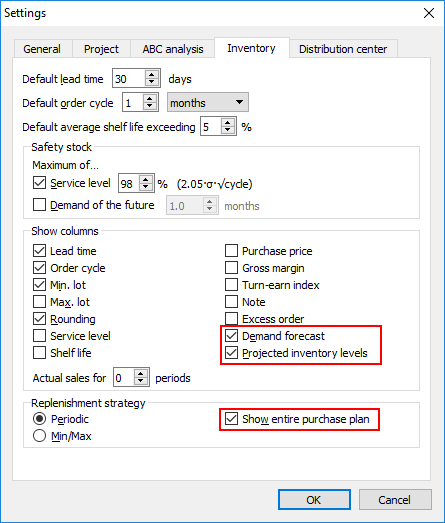As you see, the Purchase plan (and Projected inventory levels) horizon is less than the Demand forecast horizon – the Horizon parameter of Streamline (see figure below).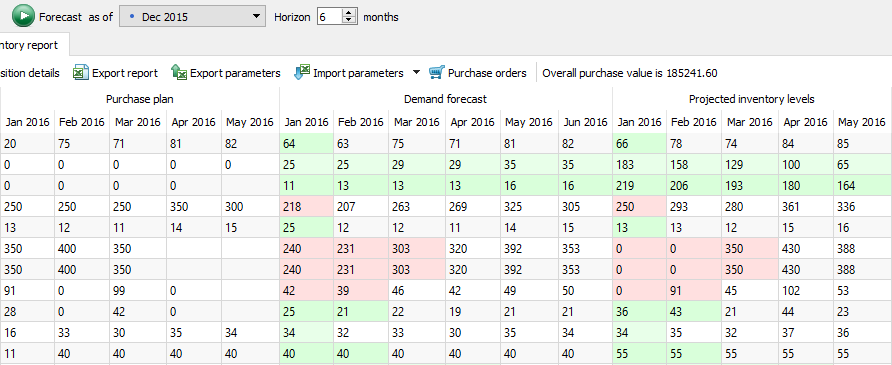The Purchase plan (and Projected inventory levels) horizon is always min(Lead time + Order cycle) – 1 data aggregation period shorter than the Demand forecast plan. Where min() means the minimal from all of the items.

### Data Required for a Purchase Plan Calculation

The Purchase plan section is calculated for each item separately. The ability to compute a purchase plan for an item depends on the availability of data, to be more precise, whether the forecast Horizon is a multiple of the lead time or not (see figure below).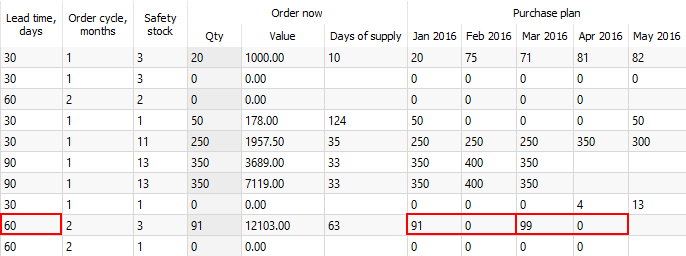As you see, Streamline can’t calculate the purchase plan for all the periods for the highlighted item (the May cell is empty). That is because the forecast Horizon doesn’t cover the last lead time period completely, consequently, Streamline can’t simulate the arrival of the last purchase order and calculate purchase plan for the periods by this arrival.

### Calculation of Purchase Plan and Projected Inventory Levels

Streamline calculates purchase plan and projected inventory levels by simulating future consumption and replenishment events.

Let’s consider the same item H8010. Recall that the order cycle and the lead time for this item are both two months.

The suggested replenishment quantities are displayed in the first period of the order cycle in the Purchase plan row of the Table. These should be ordered by the first day of the period they are shown (see figure below).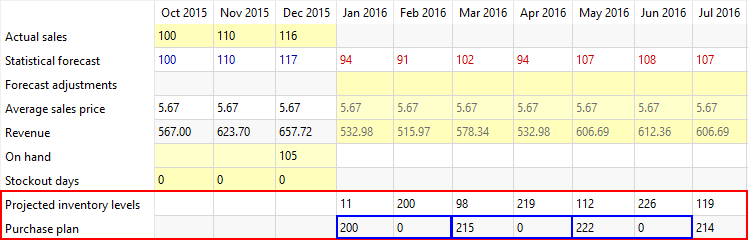Streamline recommends ordering 200 units currently (or by the beginning of January if the situation will not change). We have already explained how this suggestion was calculated. As we have a shortage (On hand is 105 units however the lead time demand is 94 + 91), Streamline expects that we will have 200 units on hand at the end of February. This quantity intended to cover the demand in March and April (see figure below).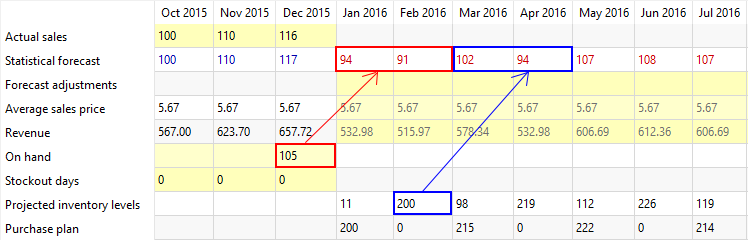Taking into account the forecast for March of 102 units, the inventory level at the end of this month is expected to be 98 units (see figure below).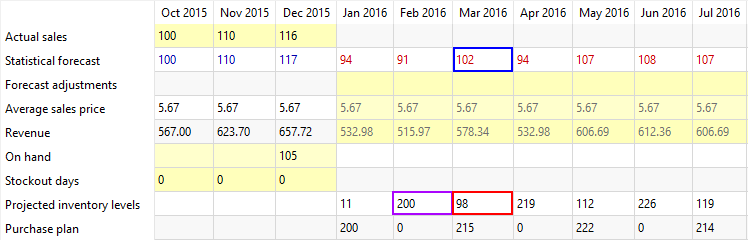To fulfill the demand for the next order cycle (May and June), Streamline suggests to order 215 units by the beginning of March (see figure below).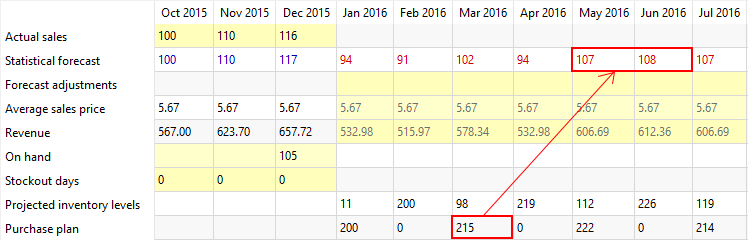This quantity is calculated using the formula (1):

Order qty = (107 + 108) + 4 + 0 – (200 - 102 - 94) = 215.

Then, taking into account the forecast for April of 94 units, order arrival of 215 units and inventory level at the end of March of 98 units, projected inventory level at the end of April is expected to be 219 units (see figure below):

Inventory level = 98 + 215 – 94 = 219.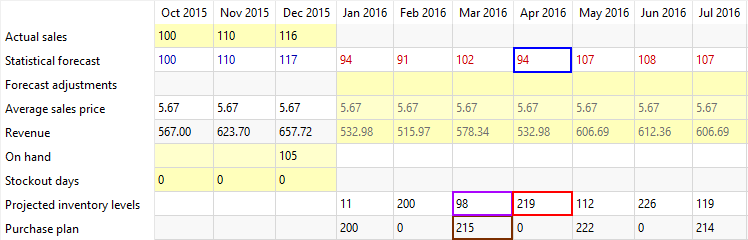And so on…

As you see, Streamline always keeps safety stock of 4 units on hand from period to period in our example.
The case we consider here is pretty simplified: there are no constraints on the lot size, and lead time and order cycle are multiple of one data aggregation period.

## Safety stock

Let’s continue examining item H8010. As you see, safety stock is only 4 units in our example (see figure below).

Why is its value so small? It’s because of the way it’s currently being calculated. To find out the method used, open the report settings by clicking the Settings button on the Inventory planning tab toolbar (see figure below).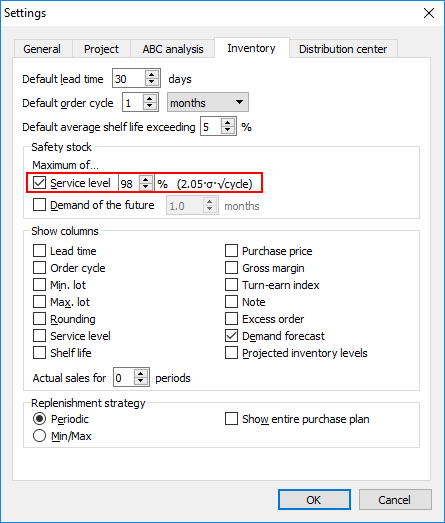We see that the calculation is based on the given Service level of 98% (meaning that we will have sufficient inventory to cover 98% of projected sales).

The exact Excel-like formula to calculate safety stock using this method is,

where:

• `Service level coefficient` is determined by the given Service level unambiguously;
• `δ` is unbiased standard deviation of the model on the learning set.

The Order cycle value is converted to the data aggregation periods before the calculation.

In our case, the model is pretty accurate - the Safety stock δ is just 1.16 (see figure below).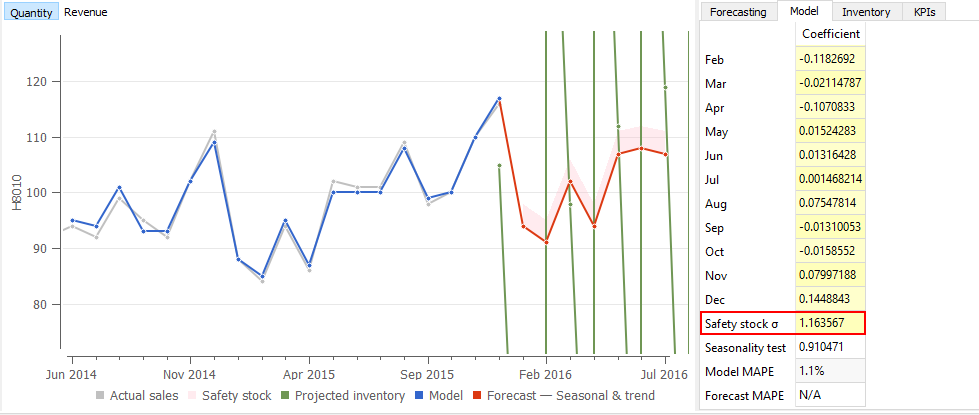Therefore, we only need to maintain a small amount of safety stock:

Safety Stock = CEILING(2.05 * 1.16 * SQRT(2)) = 4.

If you find this estimation unreliable due to a small resultant amount, you can configure Streamline to take the demand of a given number of future periods as the safety stock. To do this:

1. Go to the menu File > Settings and then go to the Inventory tab or click the Settings button on the Inventory planning toolbar.
2. Check the Demand of the future periods option.
3. Enter the number of periods.
4. Click OK (see figure below).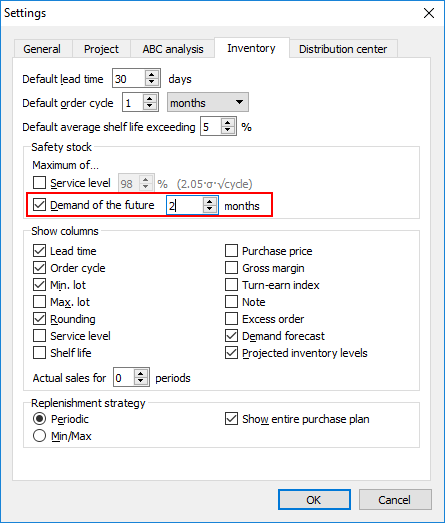The number of periods can be given as a fractional number.

As you see, Streamline has immediately recalculated the safety stock (and the entire inventory report) as we made the change. Now the safety stock is 215 units (see figure below).